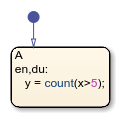# count

Number of chart executions during which condition is valid

Since R2019a

## Syntax

``count(C)``

## Description

example

````count(C)` returns the number of times that the chart has woken up since the conditional expression `C` became `true` and the associated state became active.```

## Examples

expand all

Transition out of the associated state when the variable `x` has been greater than or equal to 2 for longer than five chart executions.

`[count(x>=2) > 5]`Store the number of chart executions since the variable `x` became greater than 5.

```en,du: y = count(x>5);```## Tips

• The Stateflow® chart resets the value of the `count` operator if the conditional expression becomes `false` or if the associated state becomes inactive.

• When a chart in a Simulink® model does not have input events, the value of `count` depends on the step size. Changing the solver or step size for the model affects the results produced by the `count` operator.

• To ensure that your Stateflow chart simulates without error, do not use count in these objects:

• Continuous time charts

• Graphical, MATLAB®, or Simulink functions